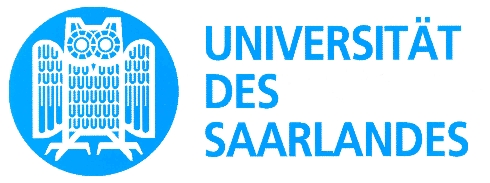# Algorithms and Randomization

## Advanced lecture course (Summer Semester 2010)

### Chinmoy Dutta, Nikolaos Fountoulakis, Anna Huber and Carola Winzen (TA)

Randomization is the power of tossing coins. Randomization and probabilistic techniques play a vital
role in the design and analysis of simple and efficient algorithms, with applications in diverse areas
ranging from combinatorial optimization and machine learning to communication networks.

This lecture course aims to introduce and explain some of the probabilistic tools and paradigms used
in randomized algorithms and their analyses. The course is designed to present the concepts by giving
numerous applications ranging from simple algorithms that find the median of a given list of numbers
to the analysis of data structures.

### Course Logistics

 Where: E1.7 Room 001 (Lectures) Tuesdays 14 - 16 (Lectures) Thursdays 18 - 20, E1.4 Room 022 This lecture is an advanced course with integrated exercise sessions (2+1). 27th July 2010 (Oral exam) Basic knowledge of algorithms and discrete probability. Most of the topics covered in the course can be found in: Probability and Computing. Randomized Algorithms and Probabilistic Analysis by Michael Mitzenmacher and Eli Upfal. Randomized Algorithms by Rajeev Motwani and Prabhakar Raghavan. Other useful and excellent study materials: Counting, sampling and integrating: algorithms and complexity, lecture notes of Mark Jerrum downloadable from here. Finite Markov Chains and Algorithmic Applications by Olle Häggström.

### Course Contents

 Lectures: See here for the list of topics covered. You are supposed to work on the problem sets individually. Problem Set 1 (Due on 4th May 2010) Problem Set 2 (Due on 18th May 2010) Problem Set 3 (Due on 1st June 2010. Deadline extended to 8th June 2010 due to midterm exams) Problem Set 4 (Due on 15th June 2010. Deadline extended to 22nd June 2010. There were typos in Exercises 4 and 5, fixed.) Problem Set 5 (Due on 29th June 2010.) Problem Set 6 (Due on 13th July 2010.)